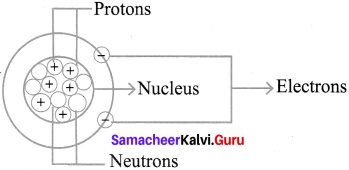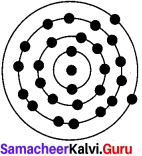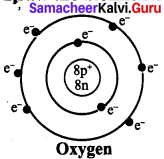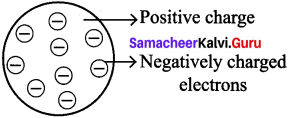# Samacheer Kalvi Books: Tamilnadu State Board Text Books Solutions

## Samacheer Kalvi 7th Science Chapter 4 Atomic Structure Notes PDF Download: Tamil Nadu STD 7th Science Chapter 4 Atomic Structure NotesSamacheer Kalvi 7th Science Chapter 4 Atomic Structure Notes PDF Download: Tamil Nadu STD 7th Science Chapter 4 Atomic Structure Notes

## Samacheer Kalvi 7th Science Chapter 4 Atomic Structure Notes PDF Download

We bring to you specially curated Samacheer Kalvi 7th Science Chapter 4 Atomic Structure Notes PDF which have been prepared by our subject experts after carefully following the trend of the exam in the last few years. The notes will not only serve for revision purposes, but also will have several cuts and easy methods to go about a difficult problem.

 Board Tamilnadu Board Study Material Notes Class Samacheer Kalvi 7th Science Subject 7th Science Chapter Chapter 4 Atomic Structure Format PDF Provider Samacheer Kalvi Books

## How to Download Samacheer Kalvi 7th Science Chapter 4 Atomic Structure Notes PDFs?

2. Click on the Samacheer Kalvi 7th Science Notes PDF.
3. Look for your preferred subject.
4. Now download the Samacheer Kalvi 7th Science Chapter 4 Atomic Structure notes PDF.

## Tamilnadu Samacheer Kalvi 7th Science Solutions Term 1 Chapter 4 Atomic Structure

### Samacheer Kalvi 7th Science Atomic Structure Textual Evaluation

I. Choose the appropriate answer :

7th Science Atomic Structure Question 1.
The basic unit of matter is __________
(a) Element
(b) Atom
(c) Molecule
(d) Electron
(b) Atom

Unit 4 Atomic Structure Answers Question 2.
The subatomic particle revolve around the nucleus is __________
(a) Atom
(b) Neutron
(c) Electron
(d) Proton
(c) Electron

Atomic Structure Class 9 Samacheer Kalvi Question 3.
__________ is positively charged.
(a) Proton
(b) Electron
(c) Molecule
(d) Neutron
(a) Proton

The atomic number of an atom is __________
(a) Number of neutrons
(b) Number of protons
(c) Total number of protons and neutrons
(d) Number of atoms
(b) Number of protons

7th Standard Atomic Structure Question 5.
__________ Nucleons comprises of
(a) Protons and electrons
(b) Neutrons and electrons
(c) Protons and neutrons
(d) Neutrons and Positron
(c) Protons and neutrons

II. Fill in the blanks

1. The smaller particles found in the atom is called _____
2. The nucleus has _____ and _____
3. The _____ revolve around the nucleus.
4. If the valency of carbon is 4 and that of hydrogen is 1, then the molecular formula of methane is _____
5. There are two electrons in the outermost orbit of the magnesium atom. Hence, the valency of magnesium _____

1. sub-atomic particels
2. Protons, Neutrons
3. electrons
4. CH4
5. 2

III. Match the following :

 1 Valency (a) Fe 2 Neutral Particle (b) Proton 3 Iron (c) Electrons in the outermost Orbit 4 Hydrogen (d) Neutron 5 Positively charged Particle (e) Monovalent

1. c
2. d
3. a
4. e
5. b

IV. True or False. If False, give the correct statement (T/F).

Structure Of Atom Class 7 Question 1.
The basic unit of an element is molecule.
(False) Correct statement: The basic unit of an element is atom.

Samacheer Kalvi Guru 7th Science Question 2.
The electrons are positively charged.
False. Correct statement: The electrons are negatively charged.

Class 7 Science Chapter 4 Notes Question 3.
An atom is electrically neutral.
True

Atomic Structure Book Back Answers Question 4.
The nucleus is surrounded by protons.
(False) Correct statement: The nucleus is surrounded by electrons.

V. Complete the analogy.

1. Sun: Nucleus, planets: ______
2. Atomic number: ______ , Mass number: number of protons and neutrons.
3. K: Potassium, C: ______.

1. electrons
2. Number of protons
3. Carbon

VI. Assertion and reason.

Samacheer Kalvi.Guru 7th Science Question 1.
Assertion : An atom is electrically neutral.
Reason : Atoms have equal number of protons and electrons.
Both Assertion and Reason are true and Reason is the correct explanation of Assertion.

Atomic Structure Class 7 Question 2.
Assertion : The mass of an atom is the mass of nucleus.
Reason : The nucleus is at the centre.
Both Assertion and Reason are true and Reason is the correct explanation of Assertion.

Atomic Structure Notes For Class 7 Question 3.
Assertion : The number of protons and neutrons is atomic number.
Reason : The mass number is sum of protons and neutrons.
Assertion is false but the reason is true statement.
Correct statement: The number of protons and neutrons is mass number.

Question 1.
Define an atom.
An atom is the smallest particle of a chemical element that retains its chemical properties.

Question 2.
Name the sub-atomic particles.
Proton, Electron, Neutrons.

Question 3.
What is atomic number?
The number of electrons or protons in an atom is called the atomic number of that atom. It is represented by the letter Z.

Question 4.
What is the characteristics of proton?
The proton is the positively charged particle and its located in the nucleus. Its positive Charge is of the same magnitude as that of the electron’s negative charge.

Question 5.
Why neutrons called neutral particles?
Neutrons are the particles in an atom that have a neutral change (no charge). They are not positive like protons. They are not negative like electrons. So they are called as neutral particles.

Question 1.
Distinguish Isotopes from Isobar.

 Isotopes Isobar Isotopes are atoms of the same element thus having same atomic number but different mass number. Isobars are such atoms which have same mass number but different atomic numbers. Thus isobars are different elements Example : Hydrogen has three isotopes protium, with deuterium, tritium atomic number 1 but mass number 1,2,3. Example : Calcium and Argon are isobars as they have atomic number 20 and 18 but their mass number is 40.

Question 2.
What are the isotones give one example.
Isotones are the atoms of different elements with same number of neutrons.
136C Neutrons = 13-6 = 7
147C Neutrons = 13-6 = 7

Question 3.
Differentiate mass number from atomic number.

 Atomic number Mass number The number of electrons or protons in an atom is called atomic number of that atom. The number of protons and neutrons present is its nucleus is called mass number of that atom. It is represented by the letter Z It is represented by the letter ‘A’. Example : Helium (He) has 2 protons and two electrons hence its atomic number will be 2. Example : Sodium (Na) has 11 protons and 12 Neutrons hence its mass number A= 11 + 12 = 23

Question 4.
The atomic number of an element is 9, it has 10 neutrons. Find the element from the periodic table. What will be its mass number?
The element is Fluorine
Its mass number A
= n + p
= 10 + 9 = 19
∴Mass number of Fluorine = 19

Question 1.
Draw the atom structure and explain the position of the sub-atomic particles. »
Structure of an atom.Position of the subatomic – particles.
Atom consist of three sub-atomic.
Particles : 1. Protons, 2. Electrons, 3. Neutrons

1. The nucleus of the atom contains the protons (positively charged) and the neutrons (no change)
2. The outermost regions of the atom are called electron shells and contain the electrons (negatively charged)
3. The protons have a positive charge while neutrons have a neutral charge.
4. Electrons circle around the nucleus of an atom, they are negatively charged.

Question 2.
The atomic number and the mass number of an element is 26 and 56 respectively. Calculate the number of electrons, protons and neutrons in its atom. Draw the structure.
Atomic number A = 26
Mass number A = 56
No. of Protons P = ?
No. of Electrons = ?
No. of Neutrons N = ?
Name of the element is Iron (Fe)
No. of proton (P) = 26
Mass number A = n+p
56 = n = 26
n = 56-26
∴ No. of neutrons n = 30
In an element No. of protons and No. of electrons is equal.
∴No of electrons = no. of protons
= 26
Structure of Iron:Question 3.
What are nucleons? Why are they so called? Write the properties of the nucleons?

1. Nucleons are sub-atomic particles (Protons and Neutrons) which are located in the nucleus of atoms.
2. Neutron and proton exist within the nucleus of the atom, together, they are called as nucleons.

Properties of Nucleons :
(a) Nucleons (Proton and Neutrons) have same mass as if they were identical particles that differ only in their electric charge.
(b) The proton carries a charge +1 and the Neutron is neutral.
(c) The size nucleon is about 1 : 6 fm (Femto meters)
(d) The force that holds the nucleus together is very short range.

Question 4.
Define valency? What is the valency of the element with atomic number 8? What is the compound by the element with hydrogen.

1. Valency is defined as the combining capacity of an element.
2. Valency of the element with atomic number : 8 Name of the element:

Oxygen : 0
Atomic number: 8
Valency : 2
Water (H2O) is the Oxide of Hydrogen and most familiar oxygen compound

X. Questions based on Higher Order Thinking Skills. :

Question 1.
An atom of an element has no electron, will that atom have any mass or not? Can atom exist without electron? If so then give example.

1. Atoms with no electron will have mass, because mass depends on number of Protons and Neutrons though it has no electron.
2. Atoms can exist without electrons, Matter is build out of neutrons, protons (+) and electrons (-). Matter becomes stable only if it is electrically neutral.
3. So atoms without electrons do exist and must have their own states (charged or uncharged transfered back and forth in their environment)
4. Example : He2+. It has 2 protons and 2 neutrons but no electrons.

Question 2.
Find what is common salt? Name the elements present in it? Write the formula of common salt. What are the atomic number and the mass number of the elements? Write the ions in the compound.

1. Common salt is mostly sodium chloride. The ionic compound with the formula NaCl, representing equal proportions of sodium and chlorine.
2. Elements present is common salt: Sodium & Chlorine.
3. Formula of common salt: NaCl Atomic number and mass number of NaCl:
Atomic number of Na = 1 Atomic number of Cl = 17 Mass number of Na = 23
Mass number of Cl = 35
4. Ions present in the compound :
Sodium ion Na+ and Chloride ion Cl

Try yourself:

Question 1.
Why are atomic numbers and mass numbers are always whole numbers ?

1. The atomic number is always a whole number because there must always be a whole number of protons in a nucleus. In other words there can never exist fractions of a proton.
2. The atomic mass number is always a whole number for the same reason. Protons and neutrons always come in single units not in fractions.

Question 2.
A sulphur atom contains 16 Protons and 16 neutrons. Give its atomic number and atomic mass number.
Atomic number of Sulphur = No.of proton sulpher
= 16
Atomic mass number of Sulphur = No. of Protons + No. of Neutrons
= 16 + 16
= 32

### Samacheer Kalvi 7th Science Atomic Structure Intext Activities

Activity – 1

Some known objects are shown, also the broken particles of the objects are shown. Name the articles or objects you see here? Also try to write What each of it made of?

4. Vessels made of Aluminum or steel

Activity – 2

Let us learn the characteristics of the subatomic particles through the following activity. Label the parts in the given diagram and answer the following.

1. The positively charged particle is ________
2. The negatively charged particle is ________
3. ________ is neutral.

1. Proton
2. Electron
3. Neutron

Activity – 3

Observe the table given above and answer the following questions.

1. I am used for breathing, without me you cannot live. Do you know me? Write my name and symbol ________
2. It is used in filling the balloons. It is a gas, identity it. What is its mass number?
3. Name the element present in banana. What is my atomic number?
4. I am found in crackers. How many protons do i have?
5. I am the most valuable element. Find who am I? Can you say my mass number?

1. Oxygen, Symbol – O.
2. Helium, Mass no. 4 Potassium, Atomic number: 19.
3. Phosphorous, No. of Protons : 15.
4. Carbon, Mass number: 12.

### Samacheer Kalvi 7th Science Atomic Structure Additional Questions

Question 1.
________ is made up of two or more atoms.
(a) Atoms
(b) Molecule
(c) Compound
(d) None
(b) Molecule

Question 2.
The average diameter of an atom is ________
(a) 10“8 m
(b) 1 m
(c) 1A°
(d) 10
(c) 1A°

Question 3.
Whose theory does not propose anything about the positive and negative charges of an atoms.
(a) J J. Thomson
(b) Dalton
(c) Rutherford
(d) Bohr
(b) Dalton

Question 4.
________ is the smallest unit used to measure small lengths.
(a) Metre
(b) Nanometre
(c) Millimetre
(d) Centimetre
(b) Nanometre

Question 5.
One Nano metre is equal to ________
(a) 108 m
(b) 10-9 m
(c) 10-5 m
(d) 10m
(b) 109 m

Question 6.
One metre is equal to ________ mm.
(a) 109
(b) 108
(c) 105
(d) 1010
(a) 109

Question 7.
Who discovered the existence of the negatively charged particles is an atom?
(a) John Dalton
(b) Rutherford
(c) Neils Bohr
(d) J.J. Thomson
(d) J.J. Thomson

Question 8.
J.J Thomson’s atom model is also called as ________
(a) Plum pudding model
(b) gold foil
(c) alpha particle
(d) none
(a) Plum pudding model

Question 9.
Protons and neutrons are called ________
(a) atom
(b) nucleons
(c) positive charge
(d) negative charge
(b) nucleons

Question 10.
Proton was discovered by ________
(a) Rutherford
(b) Goldstein
(d) J.J. Thomson
(a) Rutherford

II. Fill in the blanks :

1. ______ is the smallest particle of a chemical element.
2. The ______ are combination of atoms of various elements or the same element.
3. According to John Dalton, atom is a hard, solid, ball and it is ______
4. ________ compared an atom to a watermelon.
5. The particles that make up the atom are called _________ particles.

1. Atom
2. molecules
3. indivisible
4. J.J. Thomson
5. sub-atomic

III. Write true or false :

Question 1.
A neutron is a neutral particle.
True.

Question 2.
J.J. Thomson proposed than an atom consists of positively charged sphere in which the electrons are embedded.
True.

Question 3.
The outermost orbit of an atom can have more than 8 electrons.
(False) Correct statement : The outermost orbit of an atom contains maximum of 8 electrons.

Question 4.
Most of the space in an atom is occupied by electrons.
True.

Question 5.
Rutherford used beta particles in his scattering experiments.
(False) Correct statement: Rutherford used alpha particles in his scattering experiments.

IV. Matrix matching :

Question 1.

 Element Symbol Atomic No. i) Hydrogen a) 0 1. 11 ii) Helium b) Al 2. 8 iii) Aluminium c) He 3. 1 iv) Oxygen d) Na 4. 2 v) Sodium e) H 5. 13

1. e
2. c
3. b
4. a
5. d

Question 2.

 Particle Charge Discoverer i) Proton a) n 1. James Chadwick ii) Electron b) P 2. Rutherford iii) Neutron c) e 3. J.J. Thomson

1. b
2. c
3. a

V. Give very short answer :

Question 1.
What is atomic number?
Atomic number is the number of protons, an atom contains.

Question 2.
What is mass number of an atom?
It is the sum of protons and neutrons in the nucleus of an atom.

Question 3.
What is a neutron?
Neutron is an elementary particle that carries no charge

Question 4.
Name the 3 sub-atomic particles of an atom.
Electron, Proton, Neutron.

Question 5.
What are valence electrons?
The number of electrons present in the outermost shell of an atom are known as valence electrons.

Question 6.
What are nucleons?
Protons and neutrons reside in the nucleus of an atom and are thus called nucleons.

Question 7.
What is an electron?
It is negatively charged particle, which revolve around the nucleus of the atom is specific orbits.

Question 8.
What is the mass of a proton?
1.6726 x 10-27 kg.

Question 1.
Is the structure of the atom the same as the structure of the solar system.
Yes It is similar to the solar system. It has a core center called nucleus and it has paths called orbits around the nucleus.

Question 2.
Draw the atomic structure of Oxygen :Question 3.
what is atomic mass number? mention its formula.
Atomic mass number (A) is equal to the sum of the number of protons(p) and neutrons (n) in the nucleus.
Atomic mass or mass number = Number of Protons + Number of Neutrons

Question 4.
Define isotopes. Give example.
Atoms of the same element can have different number of neutrons. Such atoms will have same atomic number but different mass numbers. These atoms are called isotopes. For example Hydrogen has three isotopes – Hydrogen,Deuterium,Tritium

Question 5.
Define Isobars. Give example.
Atoms that have the same mass number but different atomic numbers are called Isobars.
Eg: Calcium – 40 and Argon – 40.

Question 6.
What are the limitations of Thomson’s model of an atom?
J. J. Thomson’s theory does not propose anything about the positive and negative charges of an atom. Hence , it was not able to explain many of the properties of substances.

VII. Answer the following in detail :

Question 1.
What was the model of an atom proposed by Thomson?

1. J.J. Thomson’s theory proposed that the atom has positively charged part like the red part of the watermelon and in it are embedded, like the seeds, negatively charged particles which he called electrons.
2. According to this theory as the positive and negative charges are equal, the atom as a whole does not have any resultant charge.
3. Thomson’s greatest contribution was to prove by experimentation the existence of the negatively charged particles or electrons in an atom.Question 2.
Explain the following terms.

• Proton
• Electron
• Neutron.

1. Proton (p): The proton is the positively charged particle and is located in the nucleus. Its positive charge is of the same magnitude as that of the electron’s , negative charge.
2. Neutron (n): Neutron is inside the nucleus. The neutron does not have any charge. Except hydrogen (protium), the nuclei of all atoms contain neutrons.
3. Electron (e): This is a negatively charged particle. Electrons revolve around the nucleus of the atom in specific orbits. The mass of an electron is negligible as compared to that of a proton or neutron.

VIII. Higher Order Thinking skills : (HOTS)

Question 1.
In Rutherford experiment of scattering of alpha particles, can we take foil of any other metal instead of gold?

1. Yes, we can take foil of any other metal that is highly malleable because for this experiment we need a very thin foil.
2. Gold was used because it is highly malleable, the gold foil taken was about 1000 atoms thick.

Question 2.
How Rutherford’s model is different from that proposed by J.J. Thomson?
According to Rutherford, most of the mass is concentrated is a small central part of the atom whereas J.J. Thomson thought that mass was uniformly distributed in the atom.

## How to Prepare using Samacheer Kalvi 7th Science Chapter 4 Atomic Structure Notes PDF?

Students must prepare for the upcoming exams from Samacheer Kalvi 7th Science Chapter 4 Atomic Structure Notes PDF by following certain essential steps which are provided below.

• Use Samacheer Kalvi 7th Science Chapter 4 Atomic Structure notes by paying attention to facts and ideas.
• Pay attention to the important topics
• Refer TN Board books as well as the books recommended.
• Correctly follow the notes to reduce the number of questions being answered in the exam incorrectly
• Highlight and explain the concepts in details.

## Frequently Asked Questions on Samacheer Kalvi 7th Science Chapter 4 Atomic Structure Notes

#### How to use Samacheer Kalvi 7th Science Chapter 4 Atomic Structure Notes for preparation??

Read TN Board thoroughly, make separate notes for points you forget, formulae, reactions, diagrams. Highlight important points in the book itself and make use of the space provided in the margin to jot down other important points on the same topic from different sources.

#### How to make notes for Samacheer Kalvi 7th Science Chapter 4 Atomic Structure exam?

Read from hand-made notes prepared after understanding concepts, refrain from replicating from the textbook. Use highlighters for important points. Revise from these notes regularly and formulate your own tricks, shortcuts and mnemonics, mappings etc.
Share: